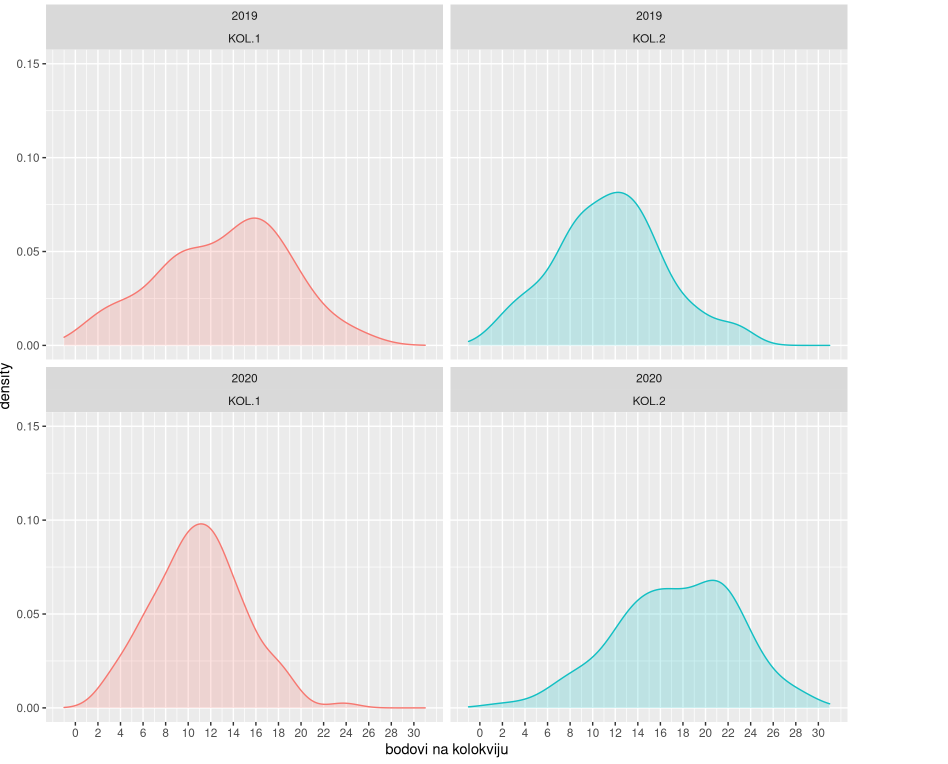# Introductory mathematical course

Course detailsIntroductory mathematical course in calculus for students of IT, engineering, economics etc. Teaching and learning strategies implemented: Flipped classroom (FC), Instruction-based learning and Project-based learning (PBL-WBL)
ECTS credits
5
Number of learners
200
Mode of delivery
Blended
Status
In planning
Course public access
Learning outcomes
Understanding

Explain the concept of primitive function and integrals of a function with one variable

Understanding

Explain the concept of the derivative of a real function of one real variable and its geometric interpretation

Applying

Apply differential calculus to find local extrema of a function with one variable and inflection points of the function.

Applying

Determine the primitive function and apply integral calculus in calculating surface area and volume.

Applying

Explain a concept of a limit and determine standard limits of functions

Analysing

Analyze an elementary function using derivatives and sketch its graph

Analysing

Analyze and solve a problem task in the area of mathematical analysis of the function of one variables

Analysing

Define elementary functions of a real variable, analyze their properties and sketch their graphs.

Creating

Create a program solution for a specific mathematical problem and present the solution in written format

##### Total Weight: 100
Course access
Contributors
• Blaženka Divjak (bdivjak@foi.hr)
• Barbi Svetec (basvetec@foi.hr)
• MIHAELA Bosak (mihaela.laljek@gmail.com)
• Damjan Klemenčić (klema123456@gmail.com)
• Marija Maksimović (mmaksimovic@uniri.hr)